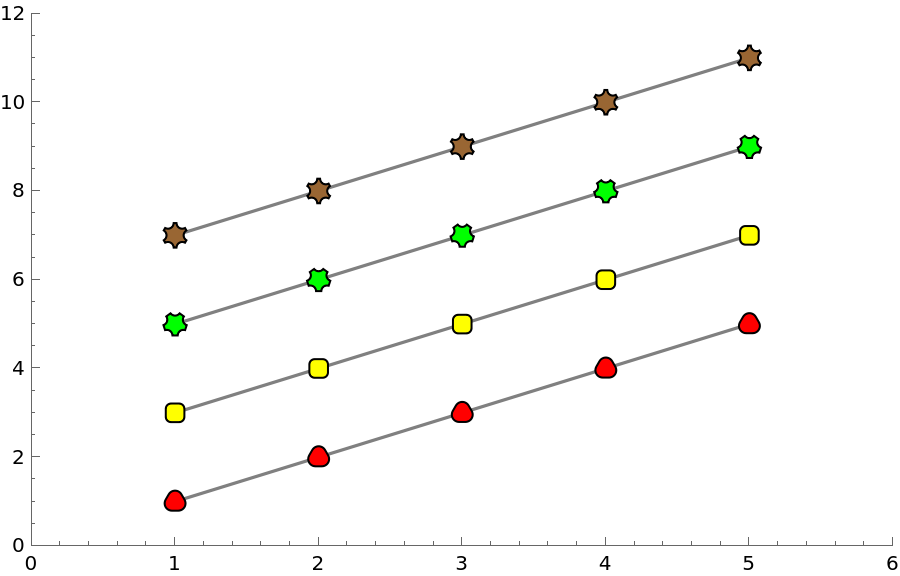Function Repository Resource:

PolygonMarker

Create markers carefully designed for creation of publication-quality plots

Contributed by: Alexey Popkov
 ResourceFunction["PolygonMarker"]["name"] returns a unit area Polygon describing the shape "name". ResourceFunction["PolygonMarker"][{p1,…,pn}] returns a unit area Polygon with shape described by points {p1,…,pn} and centroid at {0,0}. ResourceFunction["PolygonMarker"][shape, size] returns shape of the specified size. ResourceFunction["PolygonMarker"][shape, size, style] returns a Graphics object suitable for PlotMarkers where the style of shape is defined by style. ResourceFunction["PolygonMarker"][All] returns the list of names of predefined shapes.

Details and Options

The basic usage syntax is ResourceFunction["PolygonMarker"][shape,size], where shape is the name of a predefined shape or a list of 2D coordinates describing a simple polygon. The size can be given as a number or in Scaled or Offset form.
ResourceFunction["PolygonMarker"][shape,size] returns a Polygon graphics primitive that can be used in Graphics.
ResourceFunction["PolygonMarker"][shape,size,style], where style is a list of graphics directives applied to shape, returns a Graphics object which can be used as a marker for PlotMarkers.
ResourceFunction["PolygonMarker"][shape,size,style,options] returns a Graphics object with options applied.
The centroid of Polygon generated by ResourceFunction["PolygonMarker"] is always placed at {0,0} in the internal coordinate system of Graphics.
ResourceFunction["PolygonMarker"][shape,size,positions], where positions is a list of 2D coordinates, evaluates to Translate[ResourceFunction["PolygonMarker"][shape,size],positions]. It represents a collection of multiple identical copies of shape with centroids placed at positions.
With an Offset size specification, the plot marker has a fixed size specified in printer's points, independent of ImageSize.
ResourceFunction["PolygonMarker"][shape,size] returns a Polygon with area size2 in the internal coordinate system of Graphics. ResourceFunction["PolygonMarker"][shape,Offset[size]] returns a Polygon with area size2 square printer’s points. The area taken by the boundary of the returned Polygon isn’t taken into account.
ResourceFunction["PolygonMarker"][All] and ResourceFunction["PolygonMarker"][] both return the complete list of names of predefined shapes.

Examples

Basic Examples (5)

Create a polygon object of "TripleCross" type:

 In:=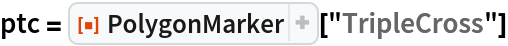Out=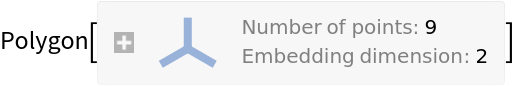The centroid of the polygon is placed at {0,0}:

 In:=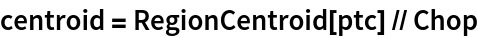Out=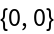Visualize the polygon and its centroid:

 In:=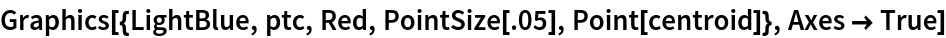Out=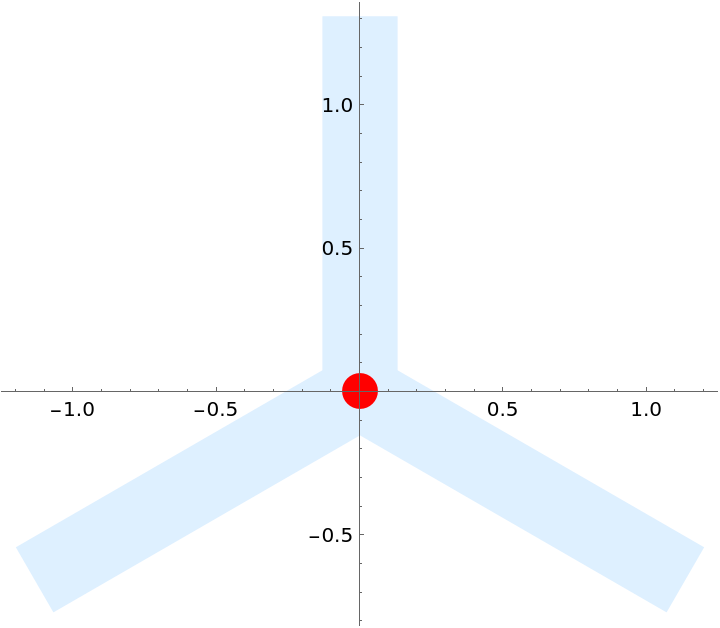Get the complete list of built-in named shapes:

 In:=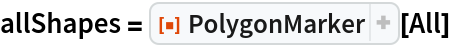Out=Make a Grid of Buttons with the shapes for easy selection:

 In:=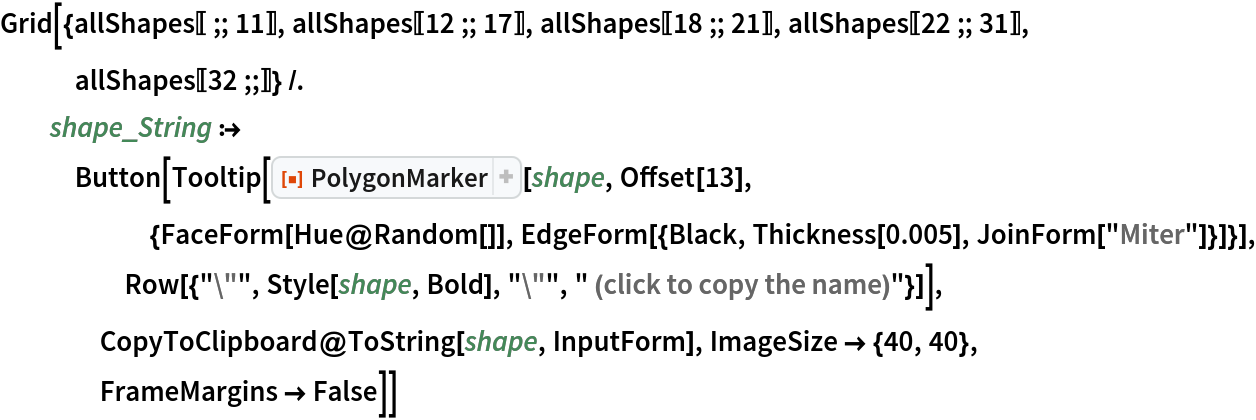Out=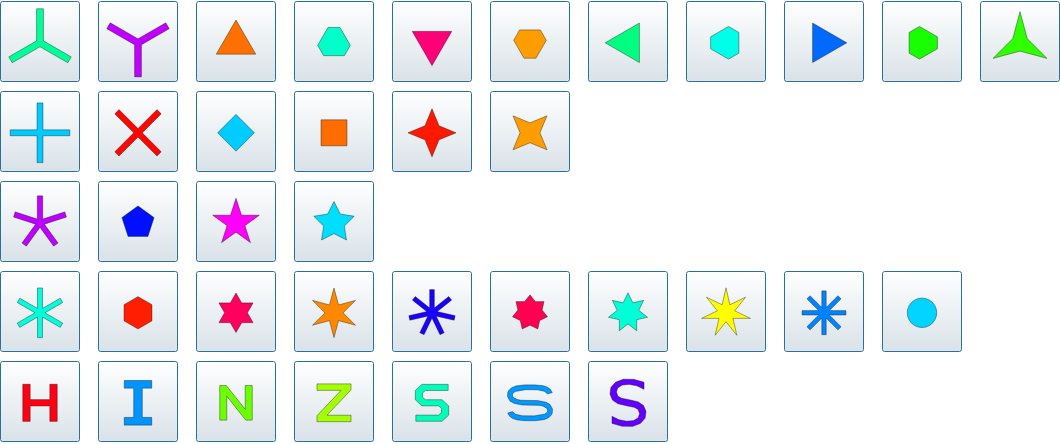Filled markers that pick up PlotStyle and PlotTheme automatically:

 In:=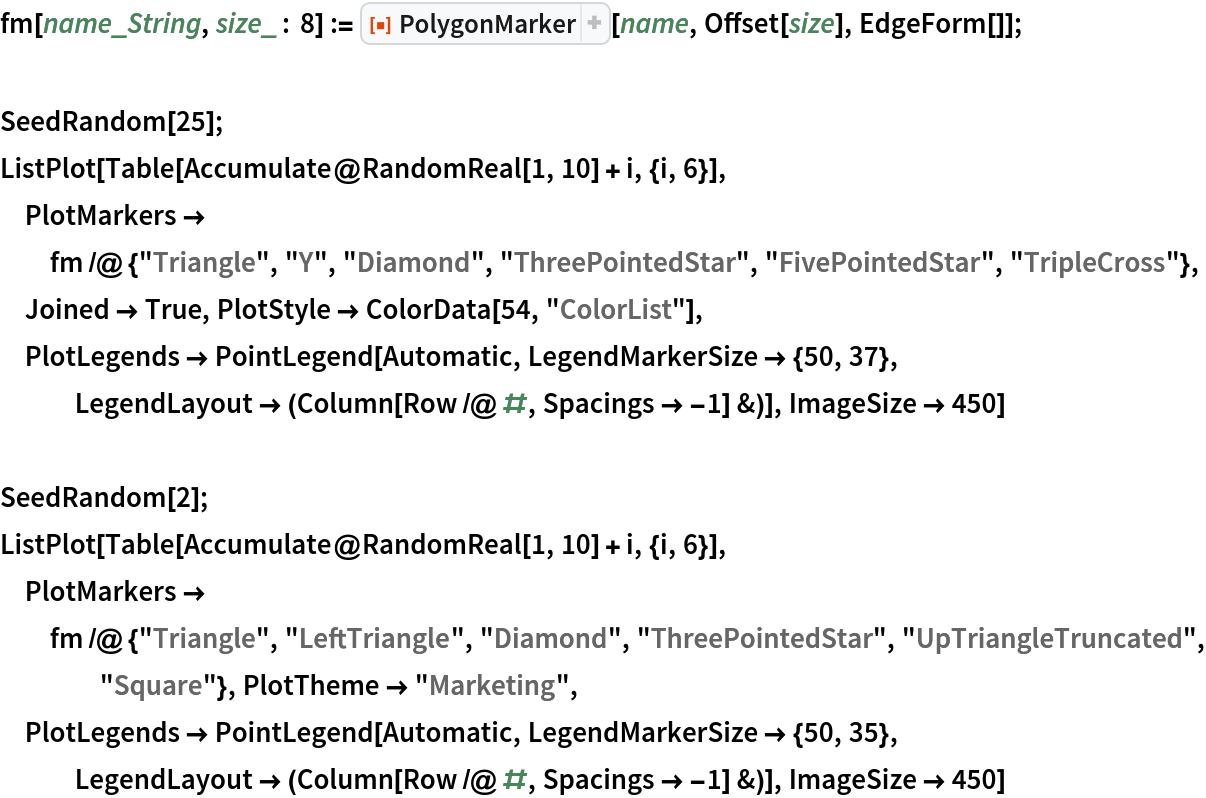Out=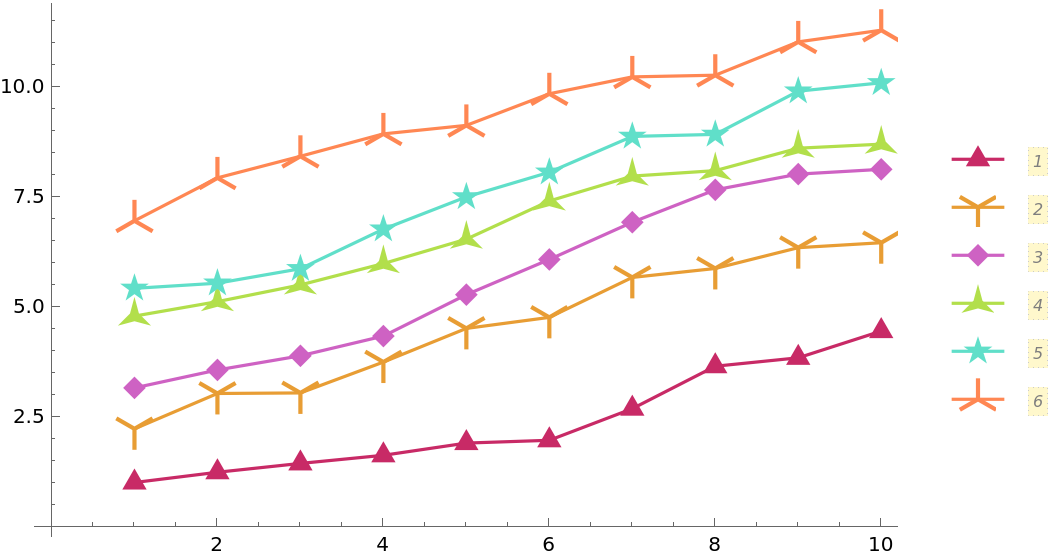Out=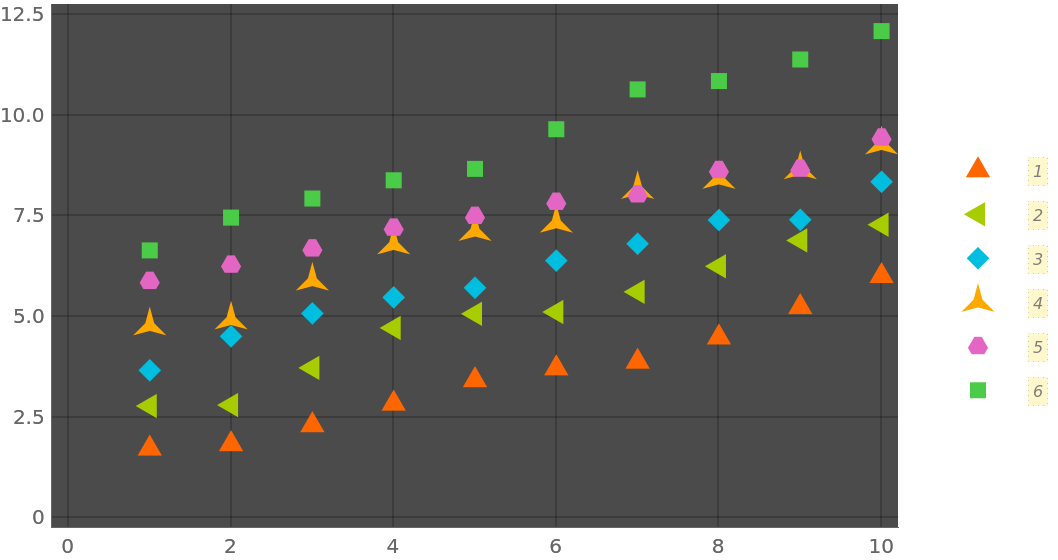Empty markers that pick up PlotStyle and PlotTheme automatically:

 In:=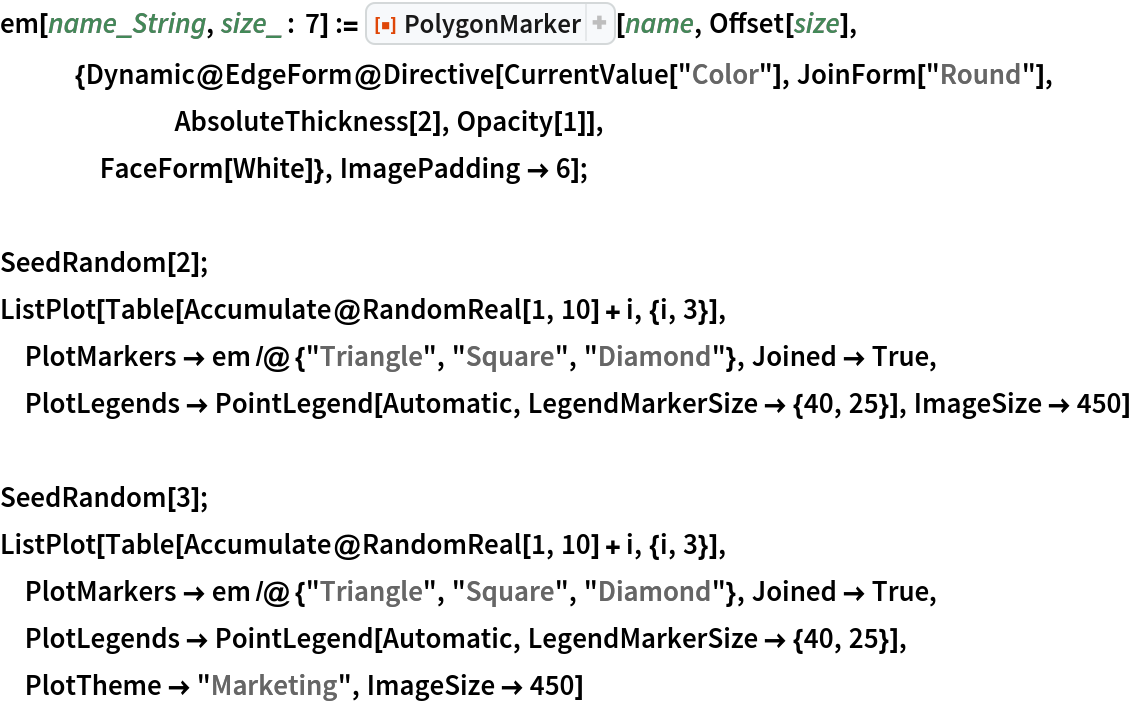Out=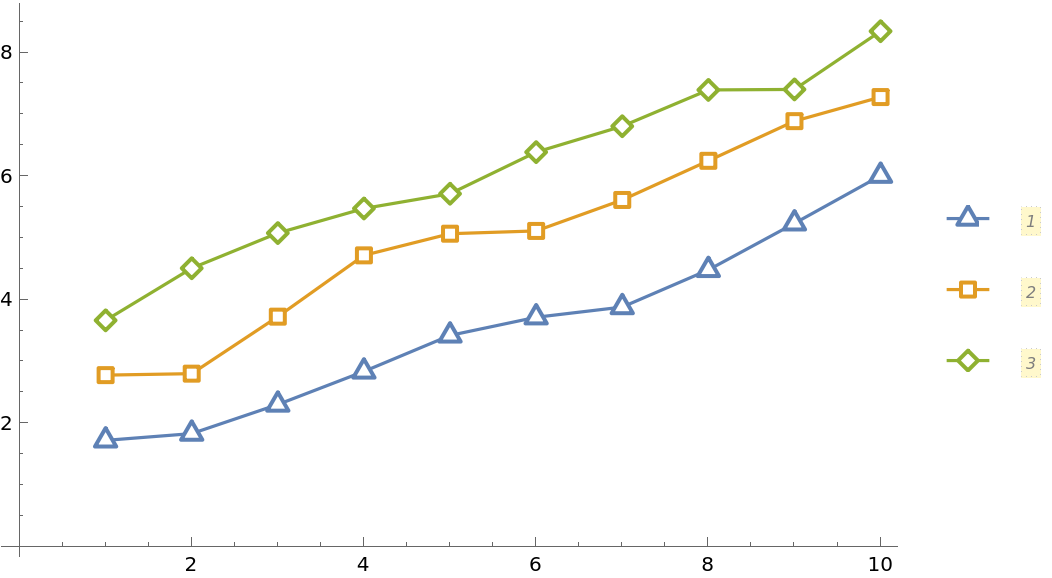Out=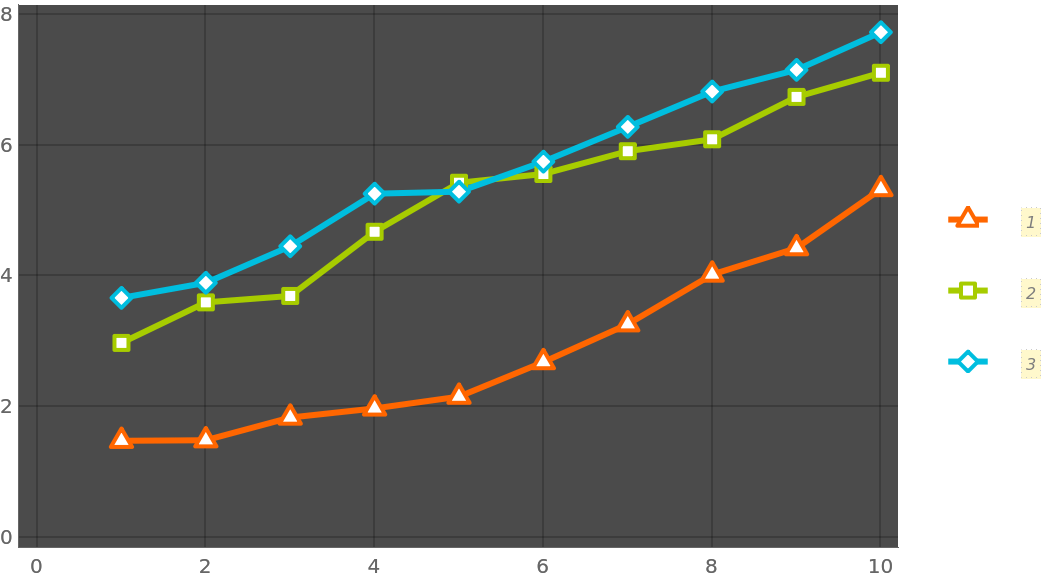Filled markers with lighter filling colors:

 In:=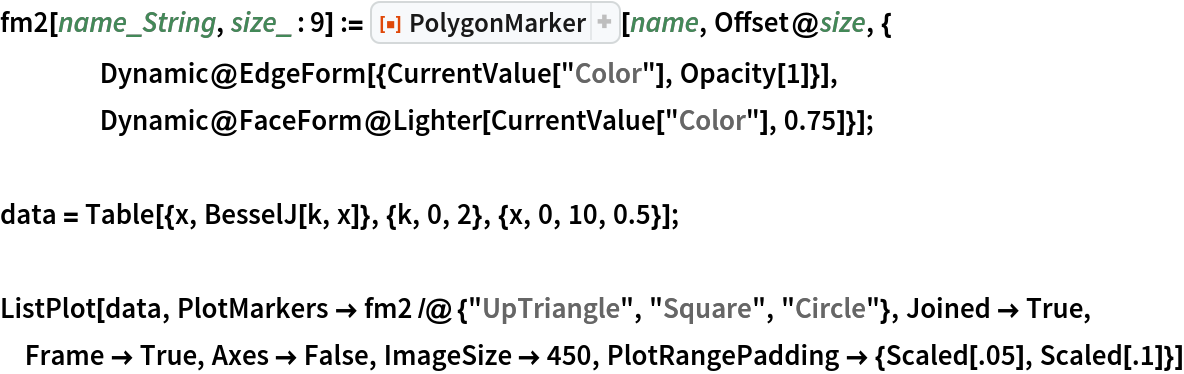Out=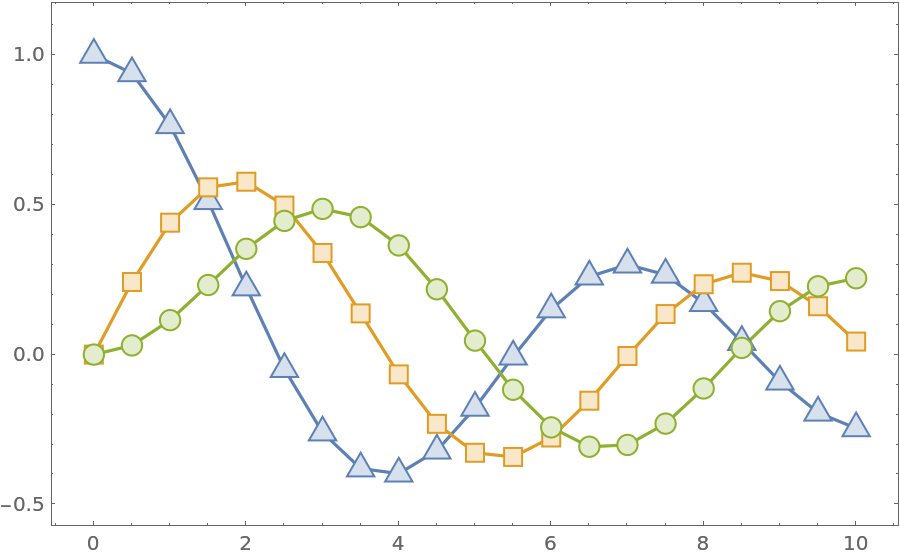Scope (3)

Use the third argument of PolygonMarker to specify the coordinate(s) where the marker should be placed:

 In:=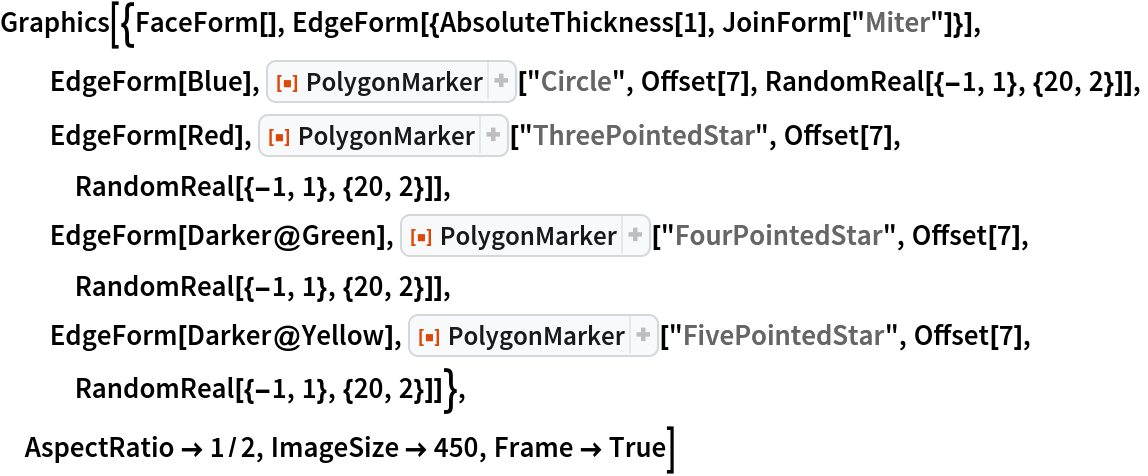Out=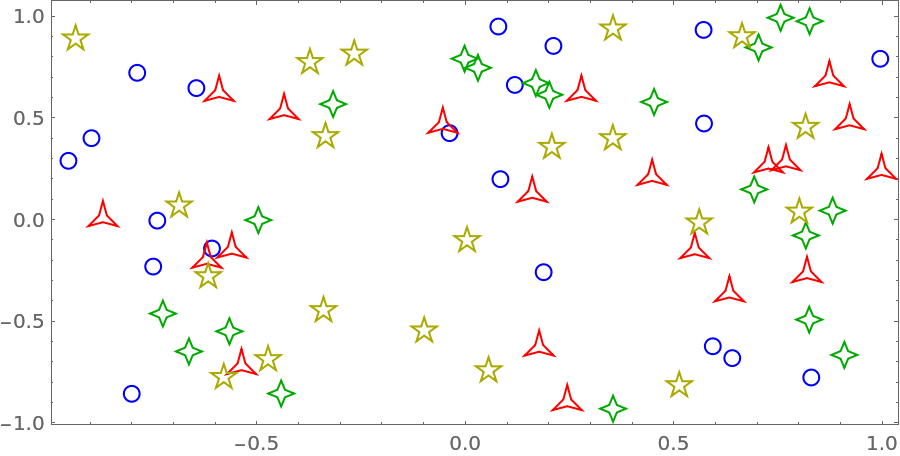Construct a list plot directly from graphics primitives:

 In:=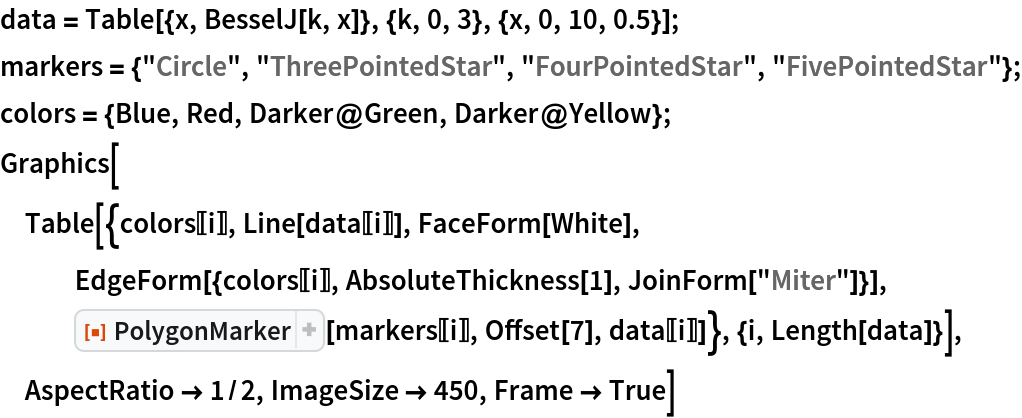Out=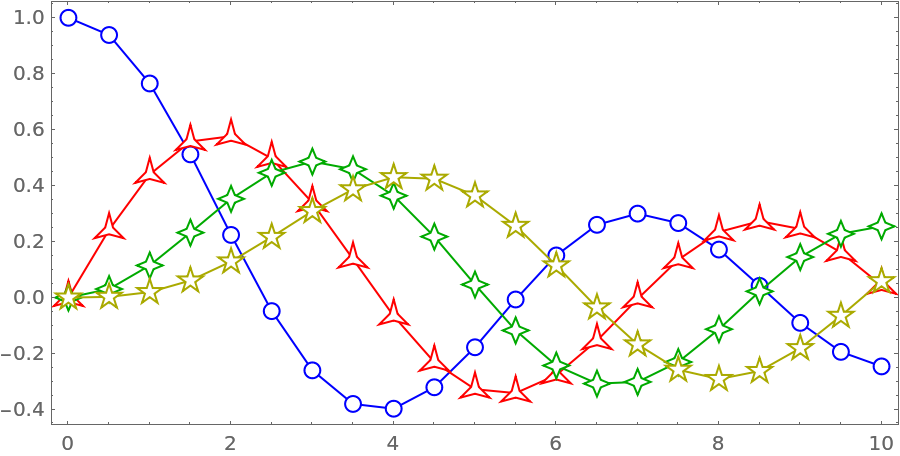Construct a custom list plot where open plot markers have transparent faces for each other (but not for the lines):

 In:=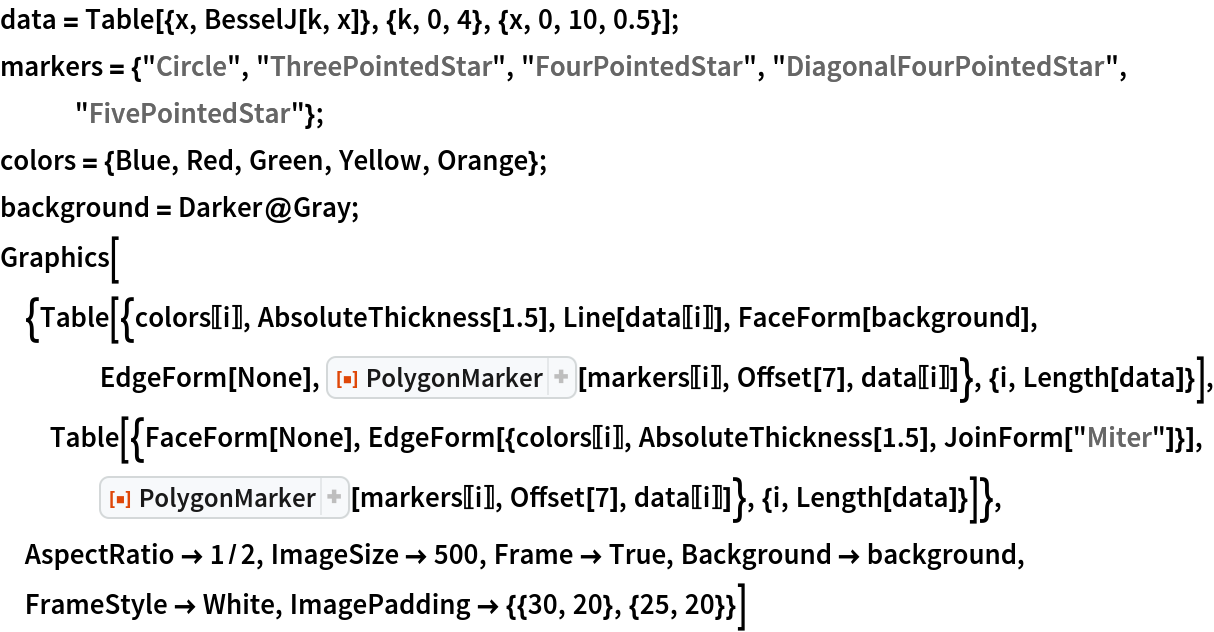Out=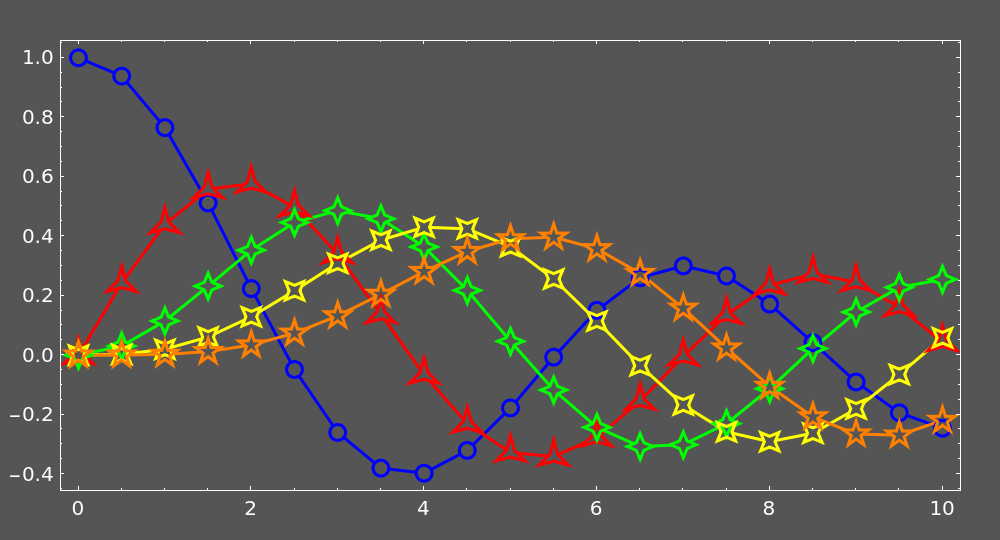Neat Examples (5)

Center markers that pick up PlotStyle and PlotTheme automatically:

 In:=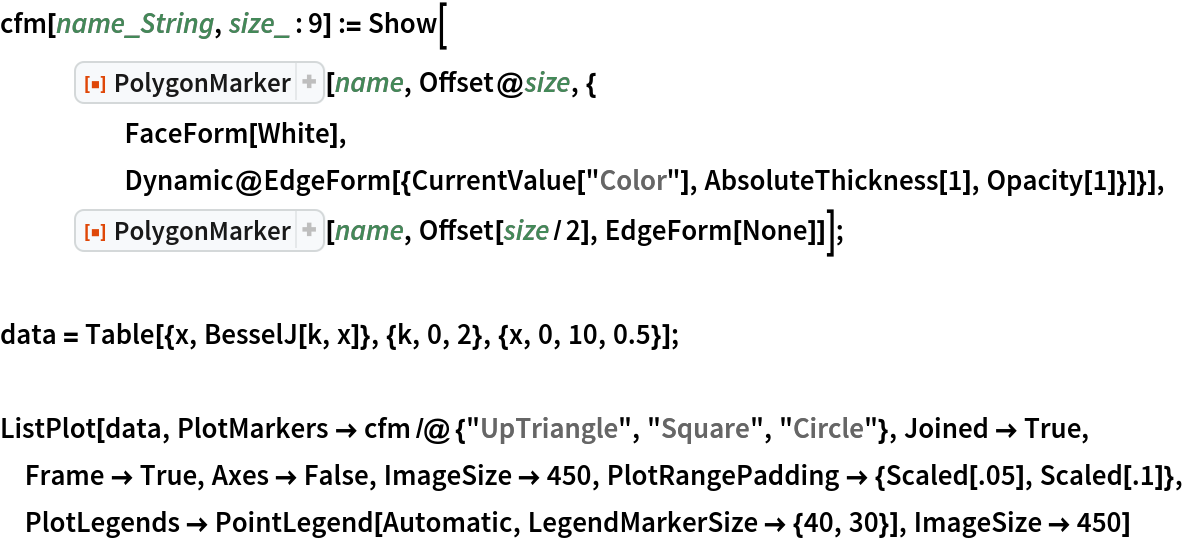Out=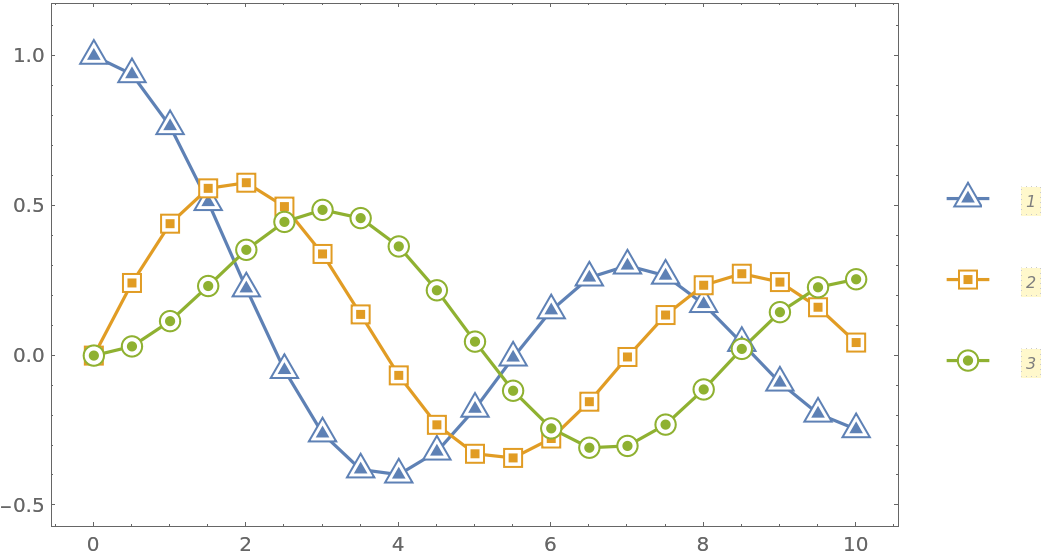Half-filled markers that pick up PlotStyle and PlotTheme automatically:

 In:=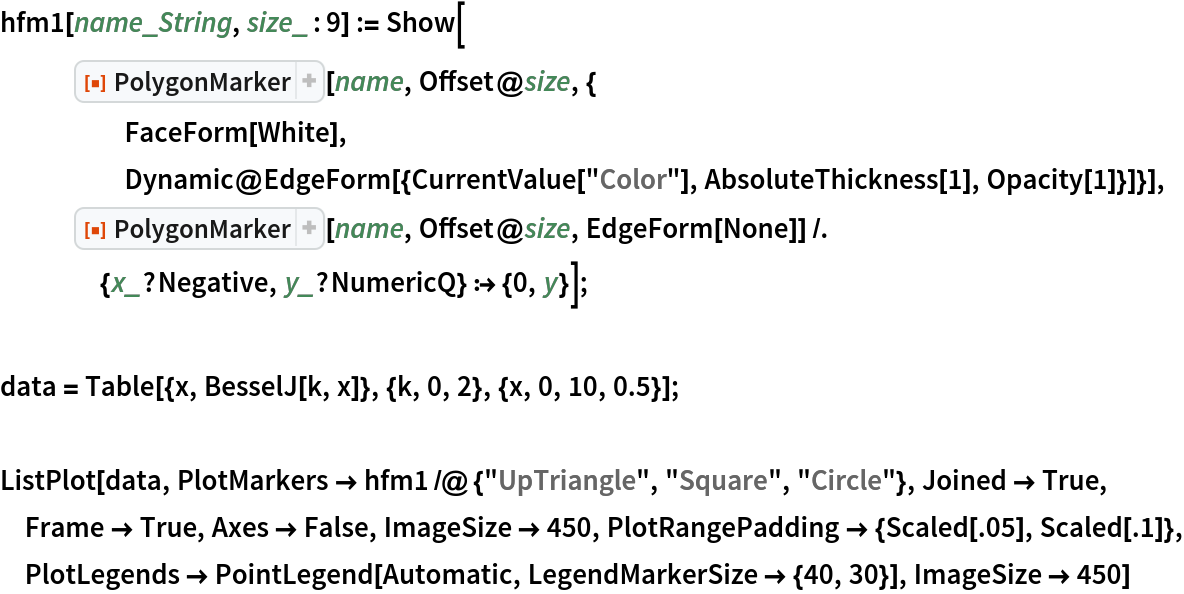Out=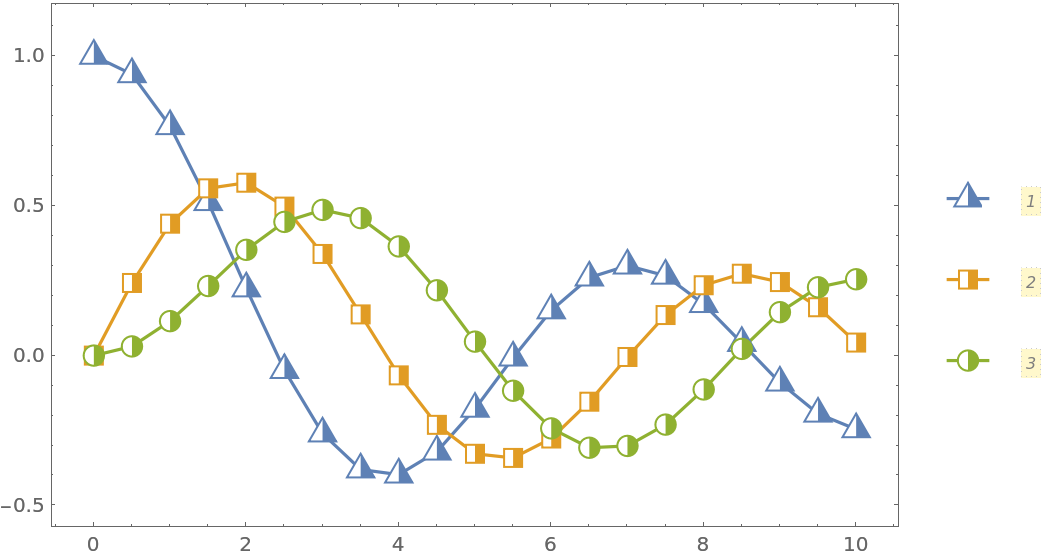In:=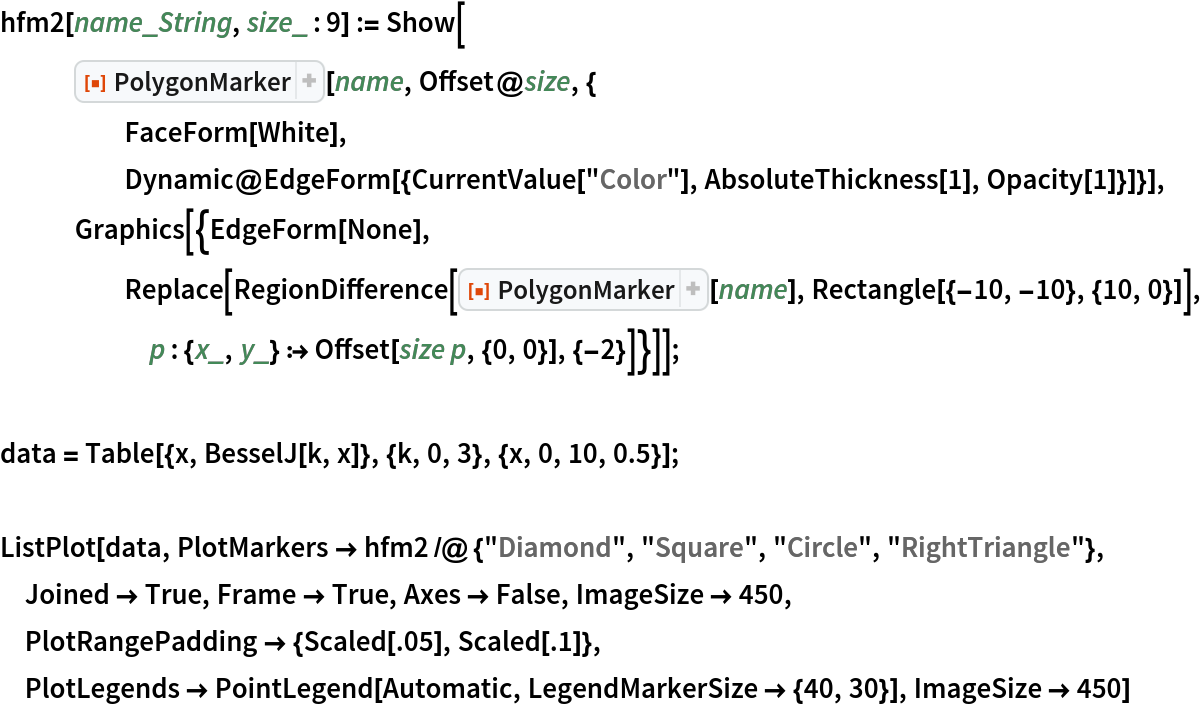Out=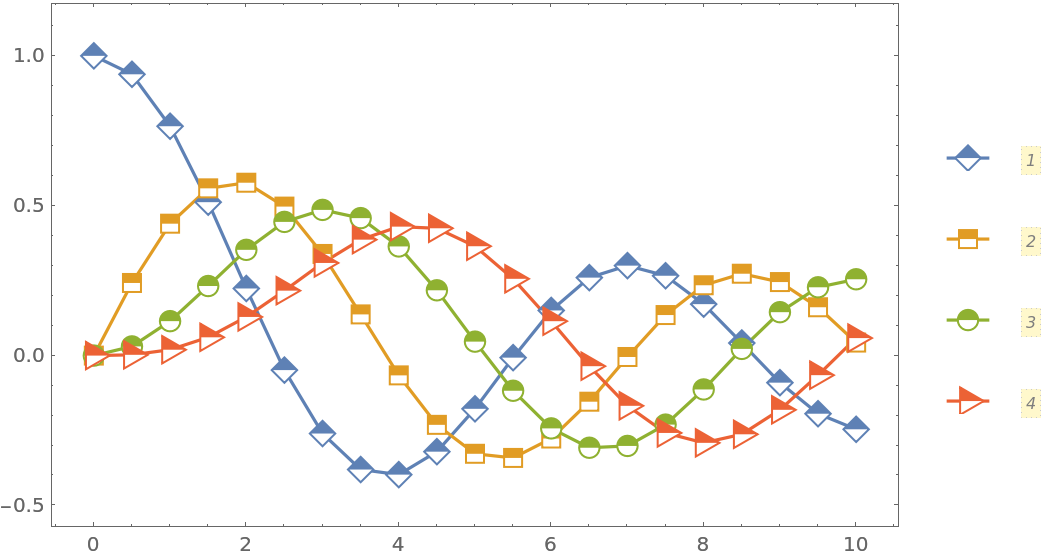In:=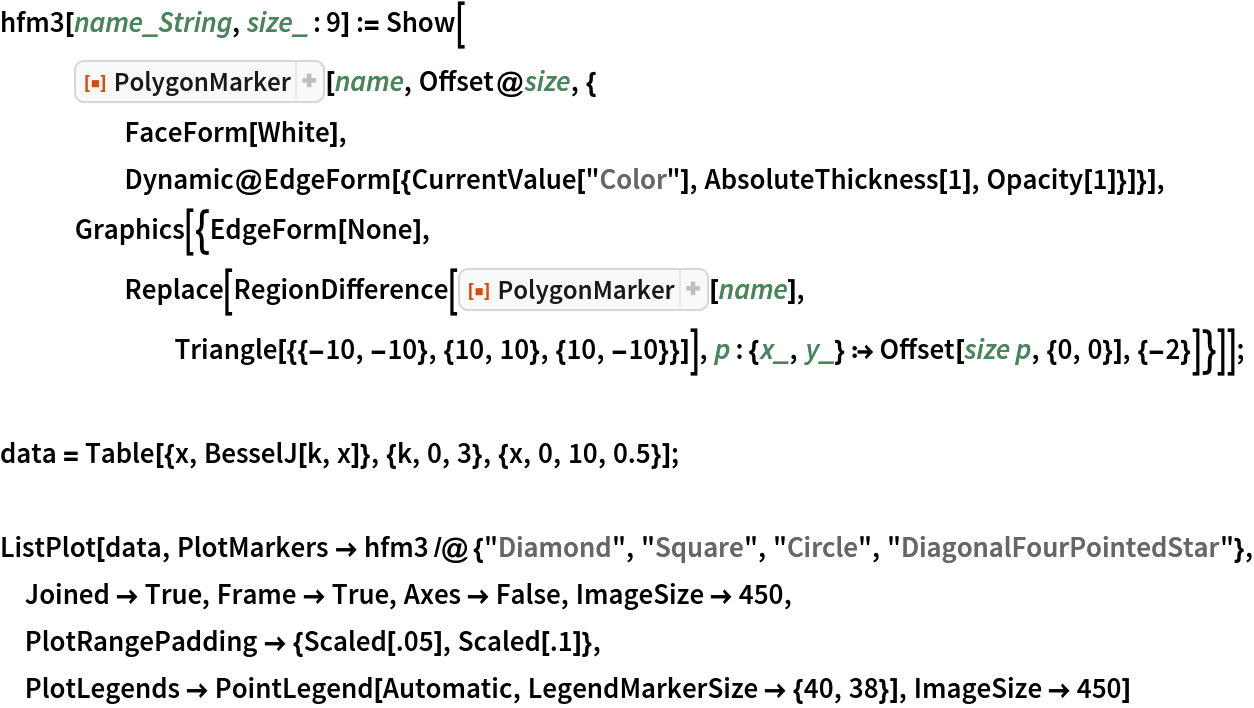Out=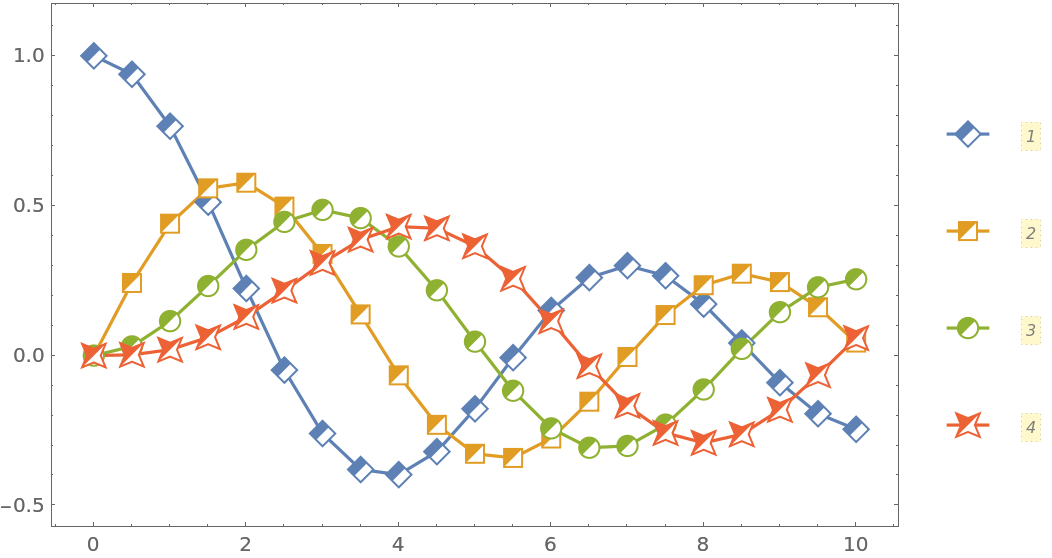Contrast markers that pick up PlotStyle and PlotTheme automatically:

 In:=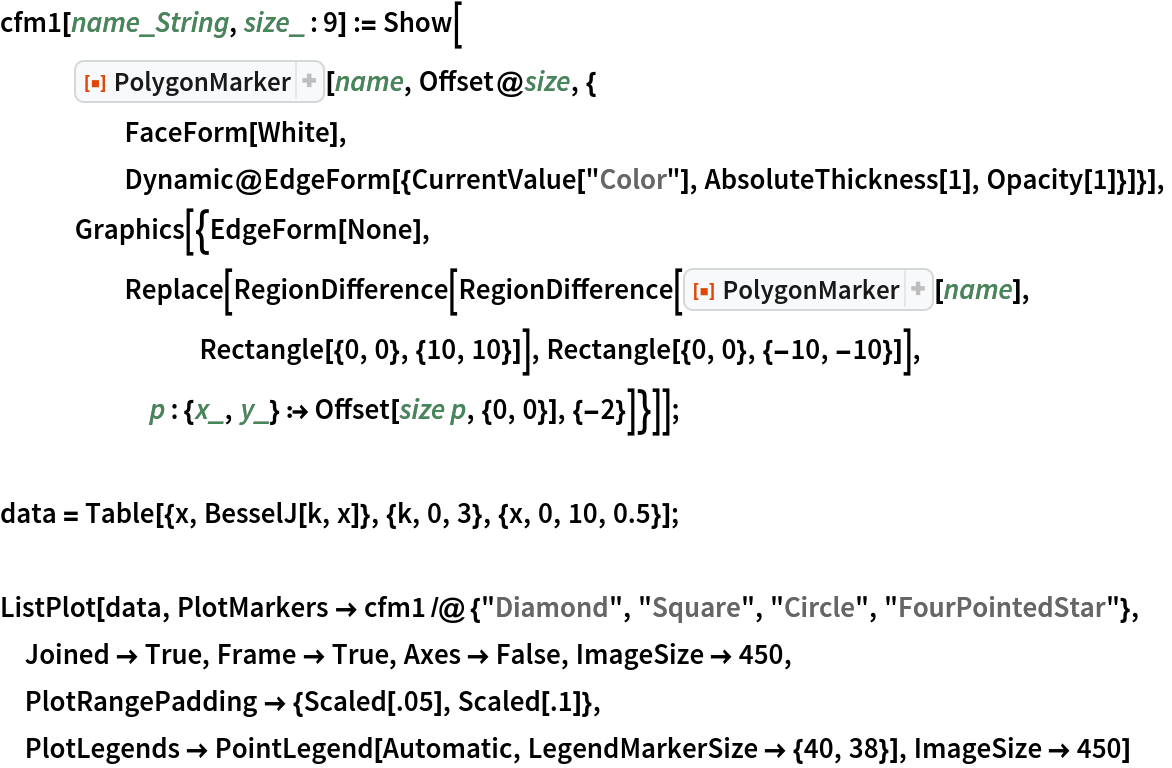Out=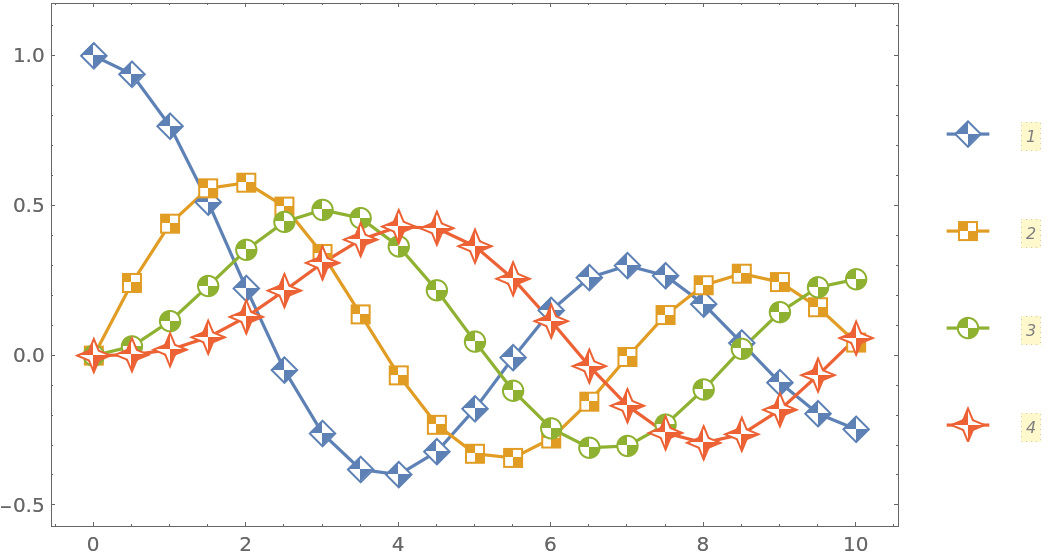In:=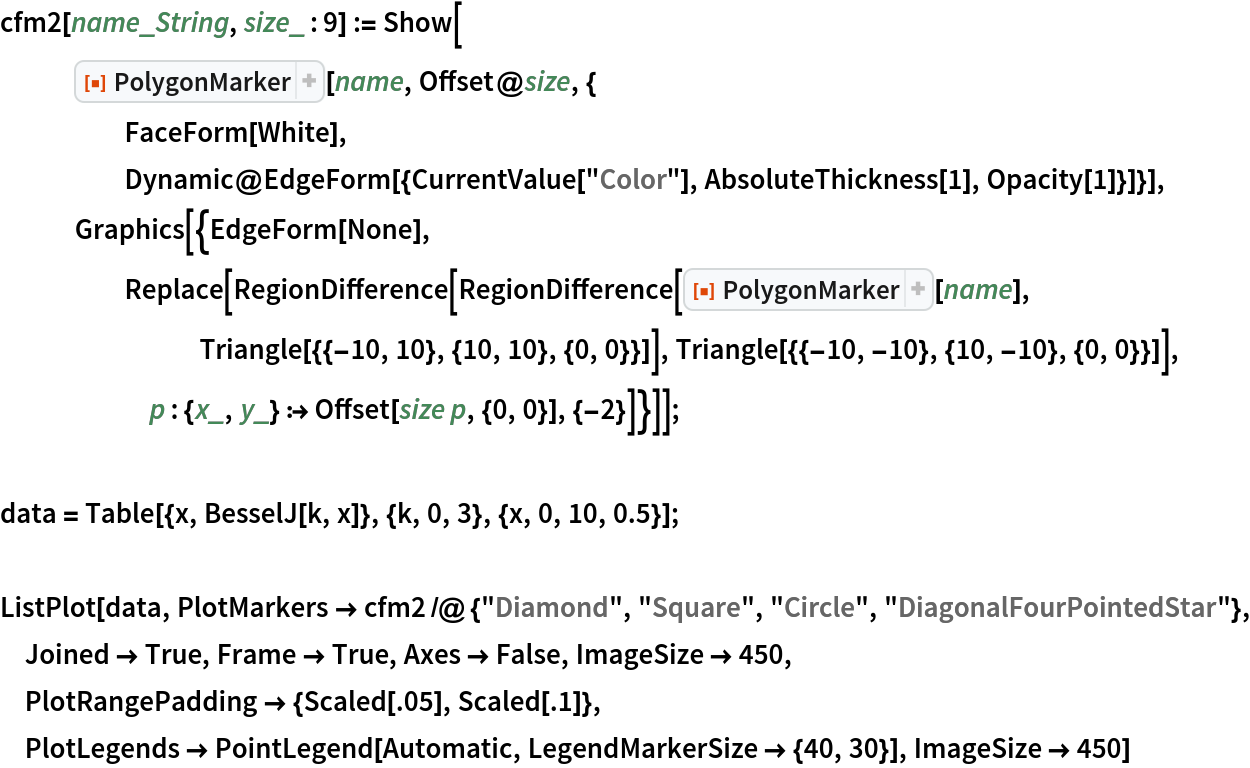Out=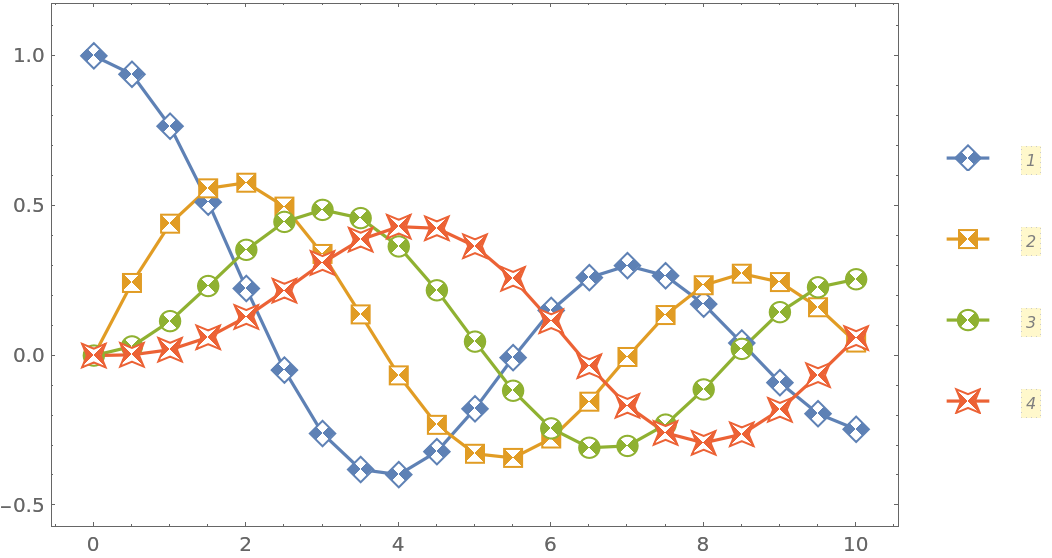Create an auxiliary function that (approximately) converts a simple glyph into a set of points suitable for PolygonMarker:

 In:=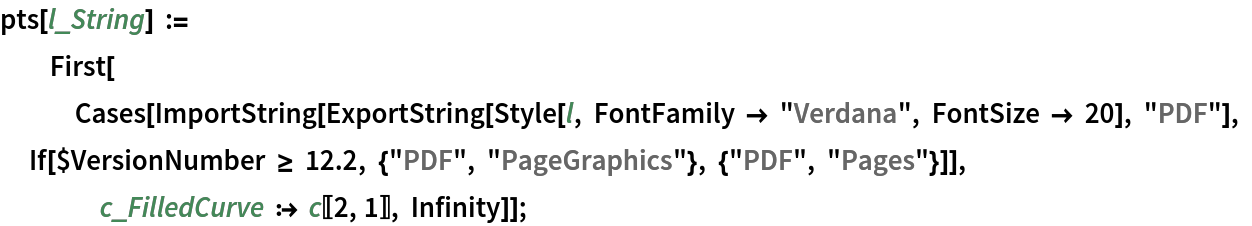Show a set of markers in use, including some created from glyphs:

 In:=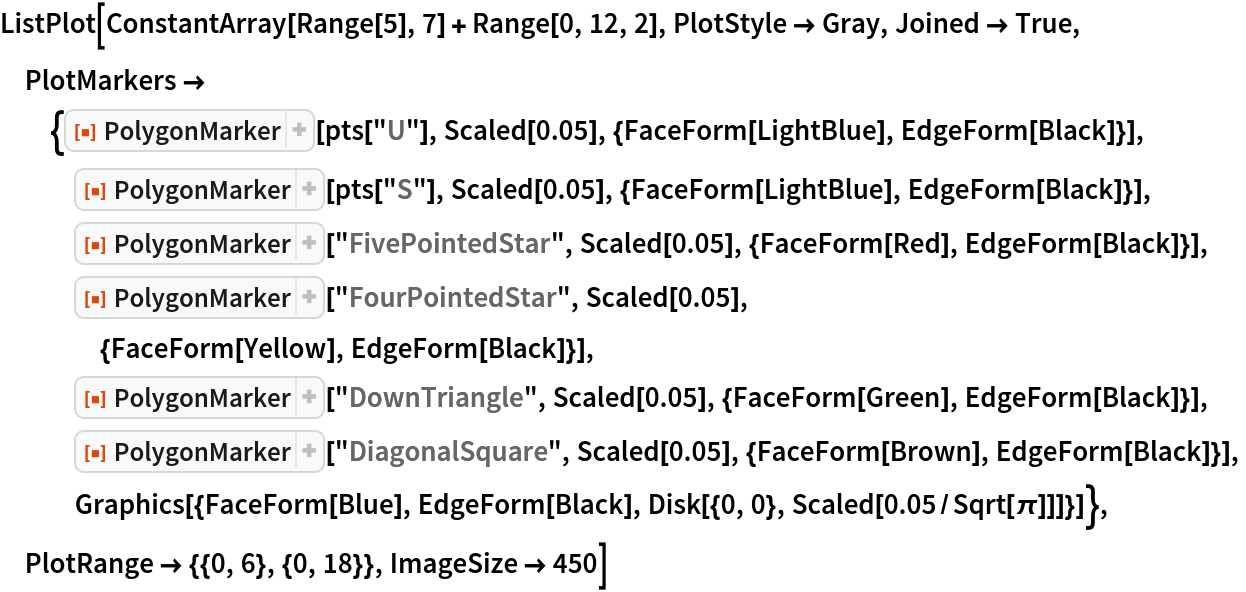Out=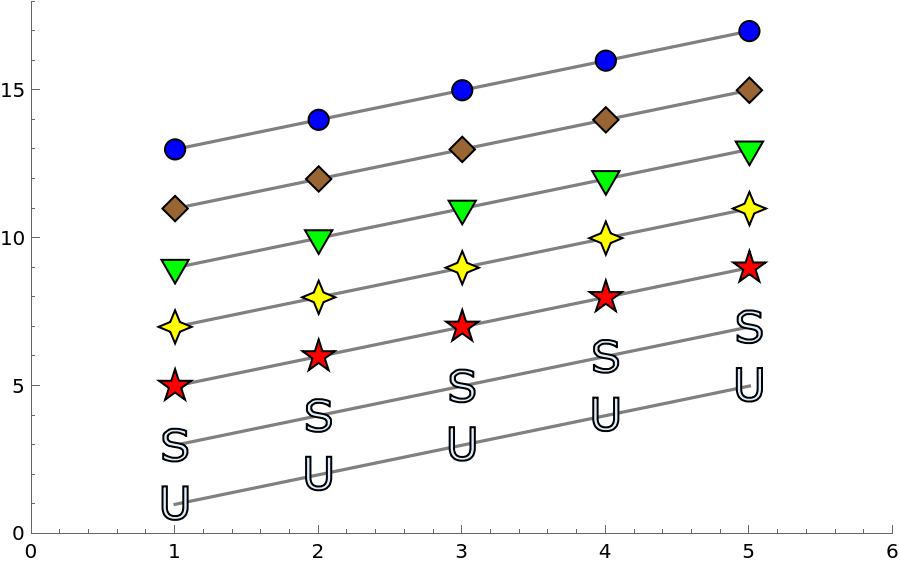Black-and-white plot, where the markers overlap considerably:

 In:=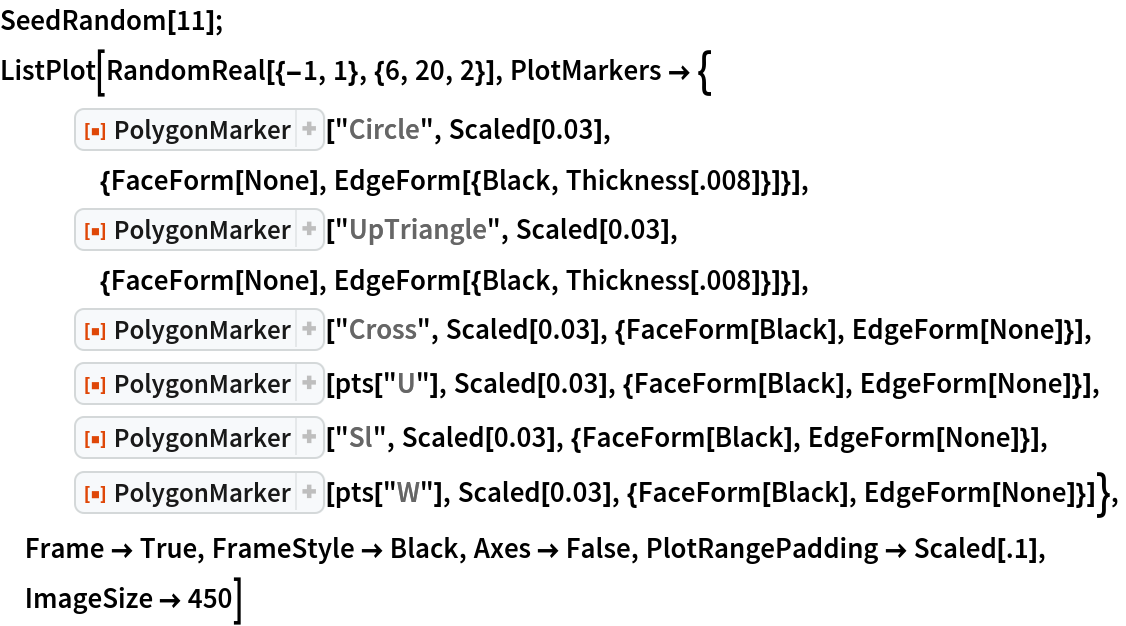Out=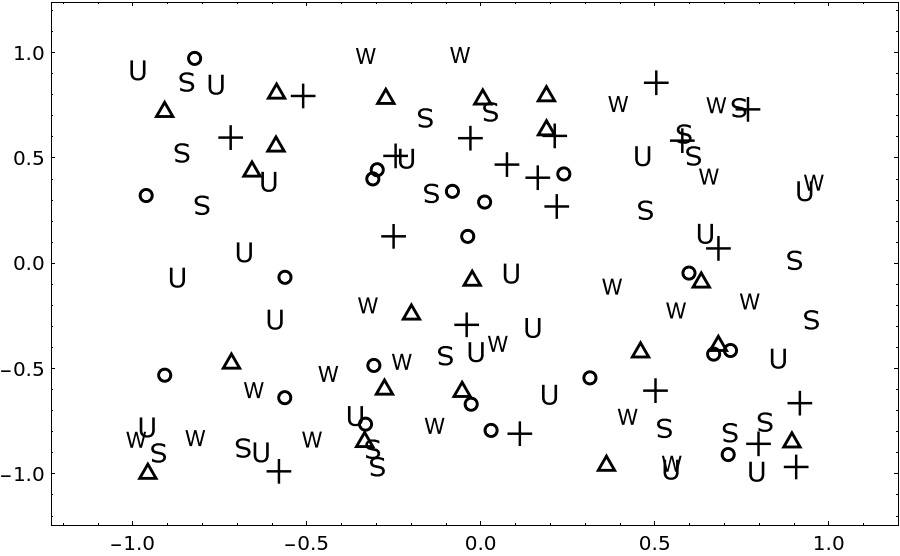Use the resource functions RoundedPolygon and NotchedPolygon to make markers:

 In:=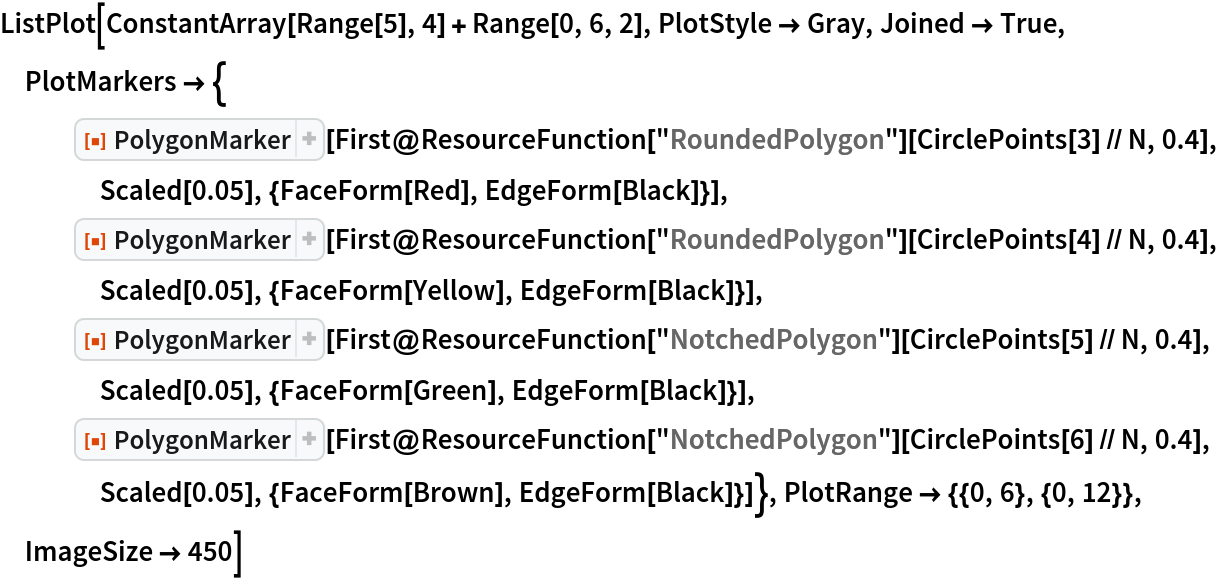Out=